Ekevad, M., and Axelsson, A. (2012). "Variation of modulus of elasticity in the tangential direction with moisture content and temperature for Norway spruce (Picea abies)," BioRes. 7(4), 4730-4743.

#### Abstract

Modulus of elasticity (MOE) in the tangential direction for Norway spruce, Picea abies (L.) H. Karst, was measured. Test samples were tested in three-point bending, and moisture content (MC) and temperature were varied between the green condition and 7% MC and between 20 °C and 80 °C, respectively. Using correction factors calculated from finite element simulations, an adjustment of measured MOE was made to the ideally tangential direction. The results show MOE and the gradients with respect to MC and temperature and how they vary with MC and temperature. The gradients are factors in gradient terms in the incremental stress-strain relation for linear elastic behaviour during load cycles where there are mechanical loads and at the same time, varying MC and temperature. The gradient terms add to the temperature and MC expansion coefficients and may be of significant size for cases with high stress, high temperature, and high MC.

Variation of Modulus of Elasticity in the Tangential Direction with Moisture Content and Temperature for Norway Spruce (Picea abies)

Modulus of elasticity (MOE) in the tangential direction for Norway spruce, Picea abies (L.) H. Karst, was measured. Test samples were tested in three-point bending, and moisture content (MC) and temperature were varied between the green condition and 7% MC and between 20°C and 80°C, respectively. Using correction factors calculated from finite element simulations, an adjustment of measured MOE was made to the ideally tangential direction. The results show MOE and the gradients with respect to MC and temperature and how they vary with MC and temperature. The gradients are factors in gradient terms in the incremental stress-strain relation for linear elastic behaviour during load cycles where there are mechanical loads and at the same time, varying MC and temperature. The gradient terms add to the temperature and MC expansion coefficients and may be of significant size for cases with high stress, high temperature, and high MC.

Keywords: Wood; Elasticity; Shrinkage; Creep; Mechano-sorption

Contact information: Luleå University of Technology, Division of Wood Science and Technology, Skeria 3, SE-931 87 Skellefteå, Sweden; *Corresponding author: Tel. +46 910 585377; Fax +46 910 585399; e-mail: mats.ekevad@ltu.se

INTRODUCTION

All the mechanical material properties of wood depend on moisture content (MC) and temperature (see, e.g., Siimes 1967; Kretschmann 1996; Green et al. 1999; Barrett and Hong 2010). This affects the stress-strain relationship for load increments where there are mechanical loading and at the same time, temperature and moisture content (MC) changes. The material parameters change during such loading increments, and the relationship must take that into consideration. The stress-strain behaviour may, in general, be non-linear and non-elastic, and the main method to model such a general relationship between stress and strain is to separate the total strain increment into two parts, a linear elastic strain component and a non-elastic strain component. With this modelling method, formulation of a linear elastic relationship between stress and strain is always needed, even if the total stress-strain relationship is non-linear and non-elastic.

A material is characterized as elastic if a body made of the material returns to its unloaded, initial configuration after it has been exposed to a loading scheme, after which all the loads on the body are returned to their original, initial values. Wood behaves as a linear elastic material for mechanical loads below a certain level and at constant MC and temperature (Wood Handbook 1999). Non-elastic behaviour is revealed as a permanent deformation for a body that is loaded and then unloaded. Non-elastic behaviour for wood is often divided into plastic, creep, or mechano-sorptive behaviour (Armstrong and Christensen 1961; Grossman 1976; Hoffmeyer and Davidson 1989; Ranta-Maunus 1990; Salin 1992; Morén 1993; Hunt 1999 and others). Models for elastic and non-elastic behaviour in combination have been discussed by many (Hanhijärvi 2000; Svensson and Mårtensson 2002; Svensson and Toratti 2002; Navi et al. 2002; Muszynski et al. 2005 and others).

In this paper, a one-dimensional linear elastic stress-strain relationship for wood is discussed, and experimentally determined material parameters for use in that relationship are presented. The tangential direction is chosen because it is the direction in wood in which shrinkage is highest and it is here judged the most important for the development of stress and strain during various wood drying processes. Data for the dependence on the modulus of elasticity (MOE) of temperature and MC and data for the gradients of the MOE with respect to MC and temperature for Norway spruce are shown. The objective of the paper is to show numerical values of MOE and gradients of MOE with respect to temperature and MC.

Existing data are scarce for MOE in the tangential direction for Norway spruce combined with dependence of MC and temperature. Gradients of MOE with respect to MC and temperature are often not explicitly shown but can in some cases be calculated from the MOE shown (Siimes 1967; Wood Handbook 1999).

EXPERIMENTAL

Theory

MOE for a hygro- and thermo-elastic material can be defined starting from a relationship between stress σ, strain ε, temperature T, and MC u. Such a relationship in one-dimensional form may be written as: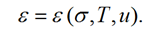(1)

For an elastic material, strain ε in a point returns to its initial (start) value after a loading scheme is applied to it, provided that all other variables return to their initial values at the end of the loading scheme. The loading scheme may consist of arbitrary variations of the variables, but the loading history does not influence the final state, which is equal to the initial state. Thus, for an elastic material, strain ε is a state function of σT, and u. Since ε is a state function, there is a perfect differential: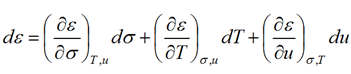(2)

The parameters in the sub-indices of the gradient expressions show which variables are kept constant during the differentiation. Now assume that the relationship between a small change of strain  and the corresponding small change of stress  when keeping T and u constant is linear. Thus dε/dσ is constant and E = E(T, u) is defined to be the MOE (not a function of σ), and the flexibility is the inverse of the MOE: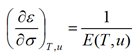(3)

This means that in addition to the elasticity assumption and definition, we have added a linearity assumption, where the expressions,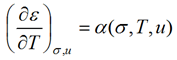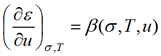(4a, 4b)

are defined as the stress-dependent thermal expansion coefficient and the stress-dependent moisture expansion coefficient, respectively. Such a type of stress-dependent thermal expansion coefficient has been discussed for metals by Rosenfield and Averbach (1956). However, since E does not depend on σ, we may achieve an expression for the dependence of α and β on σ as follows: the order of differentiation is immaterial when differentiating two times, which means that: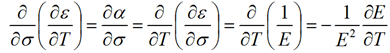(5)

Since E is not a function of σ, Eq. (5) may be integrated to,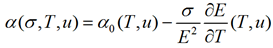(6)

where α0(T,u) is the free thermal expansion coefficient or the thermal expansion coefficient at zero stress. In the same way we get for moisture expansion: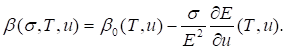(7)

The results from Eq. (6) and Eq. (7) together with Eq. (2) and Eq. (3) give a differential (incremental) form of the linear elastic constitutive behaviour of the material: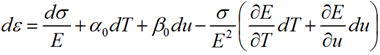(8)

The expression inside the parentheses is equal to the differential dEi.e., the change of the MOE during the increment. The last two terms are called the gradient term for temperature expansion and the gradient term for moisture expansion, respectively.

MATERIAL AND METHODS

Tests were conducted to find MOE in the tangential direction and also the dependence on MOE of MC and temperature. To get as straight growth rings as possible, the test samples had to come from the outer part of the log. Three boards from the outermost part of the logs were gathered from a local sawmill. The logs were cut from Norway spruce trees (Picea abies) which had grown in northern Sweden. The boards were planed to a thickness of approximately 21 mm before five clear wood samples with a width of 35 mm were cut from every board half. The specimens were in green condition at the start and were kept in plastic bags between drying periods to preserve MC. Three-point bending tests in a Hounsfield H25K-S bending test machine (Hounsfield Test Equipment LTD, Redhill, England) were performed for the 30 samples at three different MCs and three different temperatures, which gave a total of nine tests per specimen. To make calculations of MC possible afterwards, the specimens were weighed before each test. The dry density of the samples varied between 374 and 474 kg/m3 and the mean value was 418 kg/m3. Computed tomography (CT) images of a mid-section of the test samples are shown in Fig. 1.

Since the bending tests were non-destructive, they were stopped at a load of 60 N, which is about 20% of the estimated rupture load. To be able to use the same test span for as many specimens as possible, a test span of 110 mm was used even if the samples had a length of 170 and 180 mm. However, for a couple of specimens, the cupping deformation that evolved during the drying made a smaller span of 90 mm suitable. The first series of bending tests were performed in green condition with specimens kept in plastic bags at 20˚C (room temperature). Then the specimens (still in plastic bags) were put in an oven kept at 50˚C for about 4 hours before they were tested again. The samples were then heated once more in the same way to 80˚C, and they were tested for the third time. After that, the specimens were taken out of the plastic bags and dried at room temperature overnight. The next morning, they were placed in plastic bags again, and the second series of tests at temperatures 20˚C, 50˚C, and 80˚C were performed in the same way as described above. After further drying overnight, the third and last series of tests were conducted. The final step was to oven-dry the samples at 103˚C to establish the dry mass. MC was measured by weighing each sample before the bending test. Each weighing and bending test was performed directly after taking an individual sample from the oven, and the procedure took about 30 seconds in order to prevent cooling down during the bending test. After performing each individual test at 50°C, the sample was put back in the oven. When all samples had been tested, the oven temperature was raised to 80°C.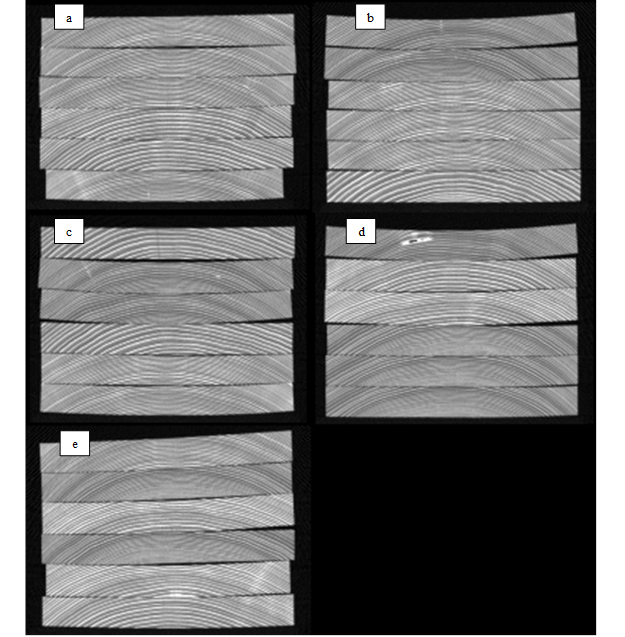Fig. 1. CT images of mid section in test samples at 7% MC. Density is shown with grey scale. a) Sample no 1 (bottom) to no 6 (top); b) Sample no 7 (bottom) to no 12 (top); c) Sample no 13 (bottom) to no 18 (top); d) Sample no 19 (bottom) to no 24 (top); e) Sample no 25 (bottom) to no 30 (top)

The test method used means that temperature T was controlled to 20°C, 50°C, and 80°C via the oven temperature settings, while the MC was uncontrolled but calculated afterward from the weights in relation to the dry mass. The testing procedure resulted in nine MOE values for each sample (one MOE for each of three temperatures and three MC values). Since MC was not controlled but instead was governed by individual properties of each sample, MC varied for each MOE for each sample.

Since the growth rings were slightly curved and not completely straight in the test samples (see Fig. 1), the measured MOE was influenced by MOE in the radial direction. Also the shear modulus influenced the measured MOE, since the slenderness ratio (span/height) was relatively low (varied between 4.3 and 5.3). In order to reduce this unwanted influence, a calibration from the test results to results for an approximately exact tangential direction was made by using correction factors on the experimental results.

A simple method was chosen as an approximate way to correct the measured values to the ideal tangential direction. The correction factors were determined by using finite element (FE) simulation results from ABAQUS (Anon 1998). Two FE models of each of the three-point bending tests were conducted, and the ratios between mid-deformation results for the two models were used as correction factors. One FE model had the actual curvature of the growth ring in the individual test sample in question and the other FE model had straight growth rings.

Both models used the same mesh and used 20-node parabolic elements. The material model was orthotropic and three-dimensional. The orthotropic axes were modelled in a cylindrical coordinate system (Ekevad et al. 2006). The following elastic material coefficients were used in all of the FE simulations: Er=424.8 MPa, Et=249.4 MPa, El=11390 MPa, Grt=34.17 MPa, Grl=729.0 MPa, Gtl=694.8 MPa, νrt=0.418, νrl=0.00707, and νtl=0.00521, where indices rt, and l stand for radial, tangential, and longitudinal direction, respectively (data from Ekevad et al. 2006). Finally, the MOE for the tangential direction was obtained as,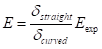(9)

where δstraight and δcurved are the FE mid-deformation results for the cases of straight and curved growth rings, respectively, and Eexp was the modulus of elasticity determined directly from the three-point bending test. The correction factors ranged between 0.85 and 0.97 for all test samples.

RESULTS AND DISCUSSION

Figure 2 shows MOE as a function of MC for all tests on all test samples at temperatures 20°C, 50°C, and 80°C. Disregarding spread, MOE was constant above fibre saturation point (FSP) at about 30% MC and was variable below FSP. This was well known from literature.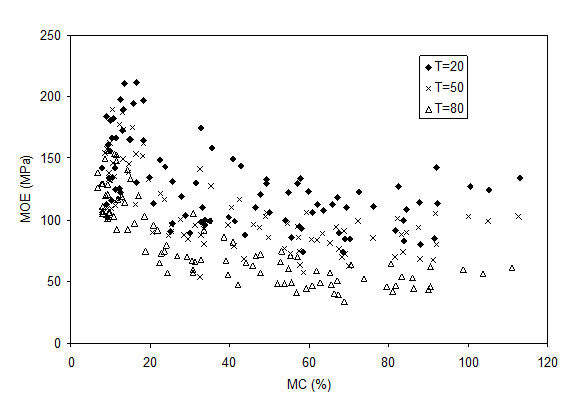Fig. 2. MOE for all test samples as a function of MC. Tests performed at temperatures 20°C, 50°C, and 80°C

Figures 3a to 3c show MOE as a function of MC for the 5 individual samples that had two MOE results for an MC<25%.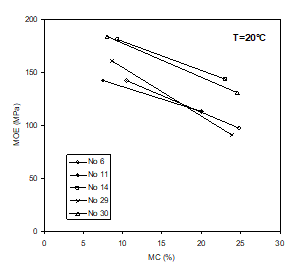3A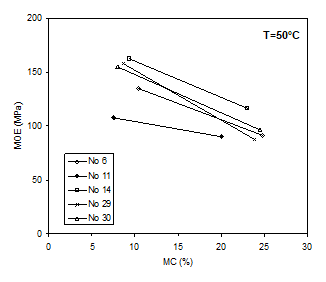3B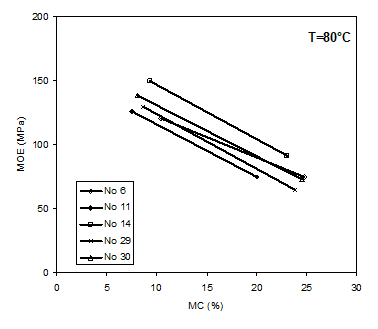3C

Fig. 3. MOE as a function of MC for 5 test samples with 2 result points below 25 % MC. No stands for sample number: a) at T=20°C, b) at T=50°C, c) at T=80°C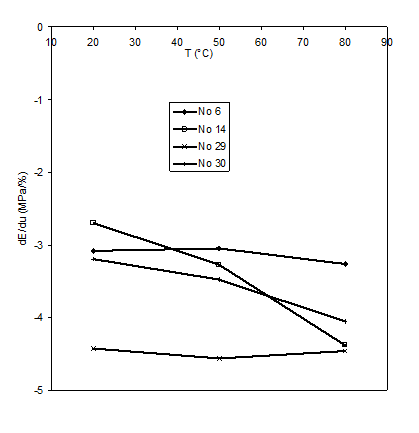Fig. 4. dE/du as a function of temperature T. Mean values for samples with 2 result points below 25 % MC. No stands for sample number

For these five samples, except for sample no. 11 (which was excluded as an outlier), dE/du below FSP was calculated and is shown in Fig. 4. The gradient dE/du below FSP in the interval 20°C to 80°C was about constant and varied in the range of -2.8 to -4.5 MPa/%. The mean value was -3.7 MPa/%.

The relative gradient (dE/du)/E for the same four samples decreased with increasing temperature, as shown in Fig. 5. Values ranged from -1.7 (dimensionless or %/%) at 20°C to -4.6 at 80°C for the individual samples. As a comparison, using MOE data in the tangential direction for spruce (mean values of tension and compression) from Siimes (1967) between a MC of 10% and FSP, the relative gradient (dE/du)/E varied between -4.4 at 20°C and -9 at 80°C.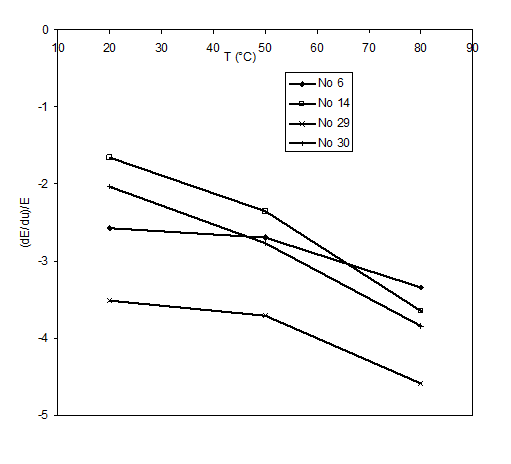Fig. 5. (dE/du)/E as a function of temperature T. Mean values for samples with 2 result points below 25 % MC. No stands for sample number

Figure 6 shows MOE as a function of temperature for all test samples. Data shown are mean values for groups of data with MC within ±2.5%. Only groups with a MC<40% are shown.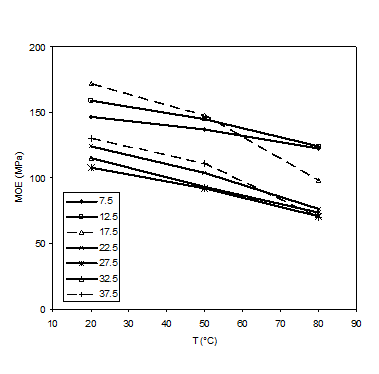Fig. 6. MOE as a function of temperature for samples with MC <40%. Mean values for 7 groups. Each group is 5% wide in MC.

Figure 7 shows the gradient dE/dT as a function of MC for temperatures 35°C, 50°C, and 65°C. The gradients at temperatures 35°C and 65°C were calculated as two-point differences between results for 20°C and 50°C and between 50°C and 80°C, respectively. The gradients at temperature 50°C were calculated as a three-point gradient using results for 20°C, 50°C, and 80°C. Linear curves fitted to the data points for each temperature and their equations are also shown. There was a small dependence of temperature on dE/dT, as can be seen from Fig. 7 and the fitted linear curves. Interestingly enough, the linear curves for different temperatures meet for MC 0% at about -0.56 MPa/°C. This indicates that water alone was responsible for the influence on dE/dT of temperature.

Figure 8 shows the gradient dE/dT as a function of MC for temperature 50°C calculated as a three-point gradient. Data are shown as mean values and standard deviation for groups with MC within ±7.5%. There are three different regions in the plot. In the first region below FSP there was a strong influence of MC on dE/dT. In the middle region between MC 30% and 90% there was a constant value of dE/dT, and in the third region, where MC is above 90%, there was again a large influence of MC on dE/dT. A hypothesis is that the first region is due to the influence of varying bound water content in the cell walls; the second region is due to saturated cell walls and only a limited amount of free water in lumen. The third region could be due to the lumen being close to being completely filled with free water.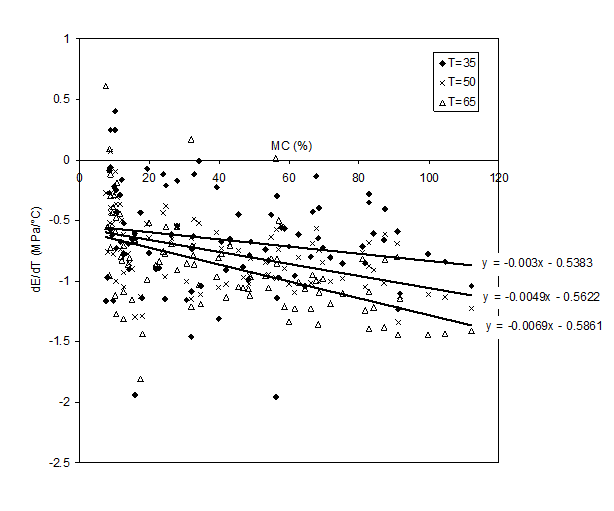Fig. 7. dE/dT as a function of MC at temperatures 35°C, 50°C, and 65°C. Straight lines fitted to data at temperature 35°C (upper straight line), 50°C (middle straight line), and 65°C (lower straight line)

Figure 9 shows the relative gradients (dE/dT)/E as a function of MC for temperatures 35°C, 50°C, and 65°C. Linear curves fitted to the data points for each temperature and their equations are also shown. For MC 0%, there was no influence of temperature and the value was 0.0038 1/°C. At 12% MC and a temperature of 50°C, we get about 0.005 1/°C. As a comparison, Wood Handbook (Anon. 1999) gives a value of MOE perpendicular to grain of 0.012 1/°C at 12% MC between 20°C and 50°C.

The first of the two gradient terms in Eq. 8, the term [-(/E2) (E/T)dT)] is an addition to the free temperature-expansion strain increment α0dT. For wood, α0dT is often neglected in comparison to β0du, but the gradient term, [-(/E2) (E/T)], may be significant, especially for high values of stress and at high temperature and high MC (which is often the case when wood dries) where the relative gradient (dE/dT)/E is large.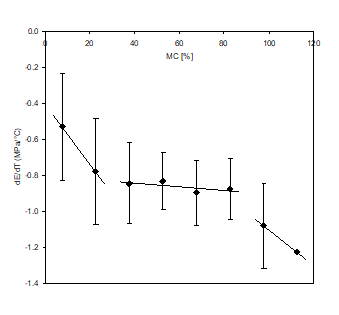Fig. 8. dE/dT as a function of MC at temperature 50°C. Mean values for 8 groups. Each group is 15% wide in MC. Standard deviation shown with error bars. Straight lines fitted to group data with MC<30%, 30%<MC<90%, and MC>90%.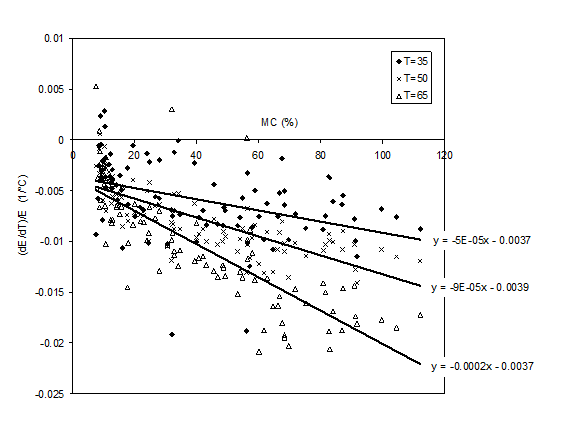Fig. 9. (dE/dT)/E as a function of MC at temperatures 35°C, 50°C, and 65°C. Straight lines fitted to data at temperature 35°C (upper straight line), 50°C (middle straight line), and 65°C (lower straight line)

The second of the gradient terms in Eq. 8, [-(/E2) (E/u)du], is an addition to the free MC expansion strain increment, β0du. The ratio [-(/E2) (E/u) / o] shows the significance of the gradient term in relation to the stress-free expansion term. In order to estimate a value for this ratio, we may set σ/E equal to 0.01 (using a reasonable value of maximum stress, e.g., from Siimes 1967), β0 equal to 0.27 (from, e.g., Wood Handbook 1999), and the relative gradient, (dE/du)/E, equal to -4.0 from this paper (Fig. 5). This gives the ratio 0.15. Using Siimes (1967) maximum value of -9 for (dE/du)/E will give an even higher ratio. These estimates show that the gradient term for moisture expansion is of significance, especially for high values of temperature and MC when drying wood.

CONCLUSIONS

1. MOE in a tangential direction is a function of both MC and temperature, and there are gradient terms in the elasticity relationship between incremental stress and strain that are of significant size, especially for situations with high stress, high temperature, and high MC (which is often the case when wood dries).

2. Even if temperature expansion is found to be relatively insignificant compared to moisture expansion, the size of the gradient term for temperature expansion may be significant.

3. For relative gradient (dE/dT)/E, there was no influence of temperature for MC 0% and the value was found to be 0.0038 1/°C. At 12% MC and a temperature of 50°C, the value was 0.005 1/°C.

4. The gradient dE/du below FSP in the interval 20°C to 80°C was about constant and varied in the range -2.8 to -4.5 MPa/%. The mean value was -3.7 MPa/%. Relative gradient (dE/du)/E decreased with temperature increase. Values ranged from -1.7 (dimensionless or %/%) at 20°C to -4.6 at 80°C for the individual samples.

REFERENCES CITED

Anon. (1998). ABAQUS Theory Manual, Version 5.8. ABAQUS Inc., Pawtucket, USA.

Anon. (1999). Wood Handbook—Wood as an Engineering Material, Forest Products Laboratory, US Department of Agriculture.

Armstrong, L. D., and Christensen, G. N. (1961). “Influence of moisture changes on deformation of wood under stress,” Nature 191, 869-870.

Barrett, J. D., and Hong, J. P. (2010). “Moisture content adjustments for dynamic modulus of elasticity of wood members,” Wood Sci. Technol. 44, 485-495.

Ekevad, M., Salin, J.-G., Grundberg, S., Nyström, J., and Grönlund, A. (2006). “Modelling of adequate pretwist for obtaining straight timber,” Wood Material Science and Engineering 1, 76-84.

Green, D. W., Evans, J. W., Logan, J. D., and Nelson, W. J. (1999). “Adjusting modulus of elasticity of lumber for changes in temperature,” Forest Prod. J. 49(10), 82-94.

Grossman, P. U. A. (1976). “Requirements for a model that exhibits mechano-sorptive behaviour,” Wood Sci. Technol. 10, 163-168.

Hanhijärvi, A. (2000). “Deformation properties of Finnish spruce and pine wood in tangential and radial directions in association to high temperature drying. Part IV. Modelling,” Holz Roh Werkst. 58, 211-216.

Hoffmeyer, P., and Davidson, R. W. (1989). “Mechano-sorptive creep mechanism of wood in compression and bending,” Wood Sci. Technol. 23(3), 215-227.

Hunt, D. G. (1999). “A unified approach to creep of wood,” P. Royal Soc. London A Mat. 455, 4077-4095.

Kretschmann, D. E. (1996). “Modelling moisture content-mechanical property relationships for clear southern pine,” Wood Fiber Sci. 28(3), 320-337.

Morén, T. (1993). “Creep, deformation and moisture redistribution during air convective wood drying and conditioning,” Doctoral Thesis, Department of Wood Technology, Luleå University of Technology, 116D.

Muszynski, L., Lagana, R., Shaler, S. M., and Davids, W. (2005). “Comments on the experimental methodology for determination of the hygro-mechanical properties of wood,” Holzforschung 59, 232-239.

Navi, P., Pittet, V., and Plummer, C. J. G. (2002). “Transient moisture effects on wood creep,” Wood Sci. Technol. 36, 447-462.

Ranta-Maunus, A. (1990). “Impact of mechano-sorptive creep to the long-term strength of timber,” Holz Roh Werkst. 48, 67-71.

Rosenfield, A. R., and Averbach, B. L. (1956). “Effect of stress on the expansion coefficient,” J. Appl. Phys. 27(2), 154-156.

Salin, J. G. (1992). “Numerical prediction of checking during timber drying and a new mechano-sorptive creep model,” Holz Roh Werkst. 50, 195-200.

Siimes, F. E. (1967). “The effect of specific gravity, moisture content, temperature, and heating time on the tension and compression strength and elasticity properties perpendicular to the grain of Finnish pine, spruce and birch wood and the significance of these factors on the checking of timber at kiln drying,” Publication 84, the State Institute for Technical Research, Helsinki, Finland.

Svensson, S., and Mårtensson, A. (2002). “Simulation of drying stresses in wood. Part II. Convective air drying of sawn timber,” Holz Roh Werkst. 60, 72-80.

Svensson, S., and Toratti, T. (2002). “Mechanical response of wood perpendicular to grain when subjected to changes of humidity,” Wood Sci. Technol. 36, 145-156.

Article submitted: June 7, 2012; Peer review completed: July 21, 2012; Revised version received and accepted: August 6, 2012; Published: August 10, 2012.